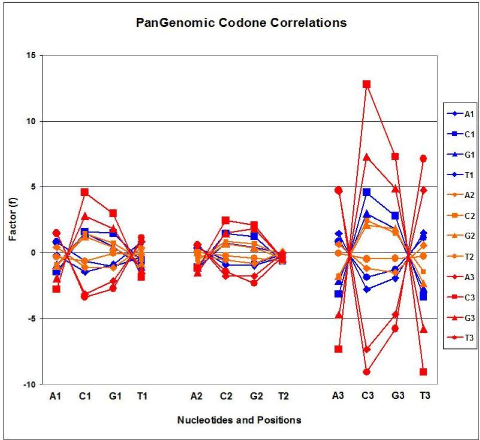Figure 4: CUF–Pan-genomic codon correlations. Codon frequencies were collated from 113 Codon Usage Frequency (CUF) Tables and the correlation coefficients (C, 64×64) were calculated. f=-log C. A–the sign was added to indicate negative correlations. The figure presents the f values between 4×4 codon letter combinations in 3×3 codon positions. Each symbol represents the mean f value (n=113). f<-2 and f>2 correspond to statistically significant correlations .